Courses

# Test: The Triangle And Its Properties - 1

## 15 Questions MCQ Test Mathematics (Maths) Class 7 | Test: The Triangle And Its Properties - 1

Description
This mock test of Test: The Triangle And Its Properties - 1 for Class 7 helps you for every Class 7 entrance exam. This contains 15 Multiple Choice Questions for Class 7 Test: The Triangle And Its Properties - 1 (mcq) to study with solutions a complete question bank. The solved questions answers in this Test: The Triangle And Its Properties - 1 quiz give you a good mix of easy questions and tough questions. Class 7 students definitely take this Test: The Triangle And Its Properties - 1 exercise for a better result in the exam. You can find other Test: The Triangle And Its Properties - 1 extra questions, long questions & short questions for Class 7 on EduRev as well by searching above.
QUESTION: 1

### How many medians a triangle can have?

Solution:

In geometry, a median of a triangle is a line segment joining a vertex to the midpoint of the opposite side, thus bisecting that side. Every triangle has exactly three medians, one from each vertex, and they all intersect each other at the triangle's centroid.

QUESTION: 2

### A/an _____________ connect a vertex of a triangle to the mid-point of the opposite side.

Solution:

A/an median  connect a vertex of a triangle to the mid-point of the opposite side.

QUESTION: 3

### How many altitudes can a triangle have?

Solution:

A triangle can have 3 altitudes

QUESTION: 4

ΔABC is right-angled at C. If AC = 5 cm and BC = 12 cm find the length of AB.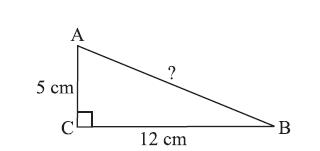Solution:

By Pythagoras property,
AB2 = AC2 + BC2
= 52 + 122 = 25 + 144 = 169 = 132
or AB2= 132. So, AB = 13 or the length of AB is 13 cm.

QUESTION: 5

Side opposite to the vertex Q of ΔPQR is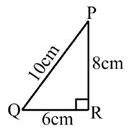Solution:
QUESTION: 6

Vertex opposite to the side RT of ΔRST is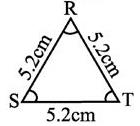Solution:

The vertex opposite to side RT is S.

Step-by-step explanation:

In the given question,

We have a triangle RST which has sides RS, ST and TR.

We know that a triangle consists three vertices and three sides each of which is particularly opposite to any one of the side of the triangle and vice-versa.

Therefore, we can see here that in the triangle RST.

The side RS is opposite to the vertex T.

The side ST is opposite to the vertex R.

and,

The side TR is opposite to the vertex S.

Hence, the correct answer will be S as side RT is opposite to S.

QUESTION: 7

In Δ PQR, PM is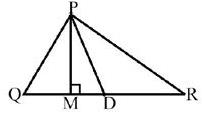Solution:

In Δ PQR, PM is altitude

QUESTION: 8

Find the value of x in the adjoining figure.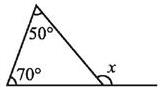Solution:

An exterior angle of a triangle is equal to the sum of the opposite interior angles

xo = 70o + 50o

xo = 120o .

So option C is the correct answer.

QUESTION: 9

Find the value of x.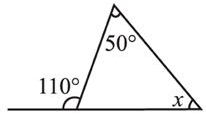Solution:

An exterior angle of a triangle is equal to the sum of the opposite interior angles

So in the given figure

110o = 50o + x

110o - 50o = x

60o = x

So option B is correct answer.

QUESTION: 10

Find the value of x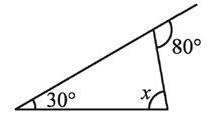Solution:

An exterior angle of a triangle is equal to the sum of the opposite interior angles

So in the given figure

80o = 30o + x

it implies x = 50o

QUESTION: 11

If the angles of a triangle are in the ratio 4:5:9. Find all the angles of a the triangle

Solution:

The sum of the angles of a triangle is 180 degrees. As the ratio of the angles of the triangle is 4:5:9 they can be taken to be 4x, 5x and 9x. 4x + 5x + 9x = 180

=> 18x = 180

=> x = 10

This gives the angles of the triangle as 4*10=40,

5*10=50 and 9*10=90 degrees.

QUESTION: 12

The triangle whose lengths of sides are 3 cm, 4 cm, 5 cm is a

Solution:

a2+b2=c2

we find 32+42=52

9 + 16 = 25

So, these sides also cause it to be a right triangle.

QUESTION: 13

One of the angles of a triangle is 110° and the other two angles are equal what is the measure of each of these equal angles

Solution:

One angle of the triangle = 110°
Let the other two angles each be x
Sum of all the angles of a triangle = 180°
x + x + 110 = 180
2x = 180 - 110
2x = 70
x = 35°
The other two angles are 35°, 35°.

QUESTION: 14

The measure of three angles of a triangle are in the ratio 5:3:1.find the measure of this angles

Solution:

Let the angles be x
sum of the angles of a triangle=180

5x+3x+x=180
9x=180
x=20
angle I = 5x = 5*20 = 100
angle II = 3x = 3*20 = 60
angle III = x = 20

QUESTION: 15

The altitude and median be same for a which triangle?

Solution:

Since the sides of the isosceles triangle are equal the line drawn from vertex to middle of the opposite side which is median is perpendicular. And altitude is also perpendicular . So both are the same in the isosceles triangle.